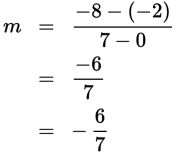# SAT Math Multiple Choice Question 516: Answer and Explanation

### Test Information

Question: 516

6.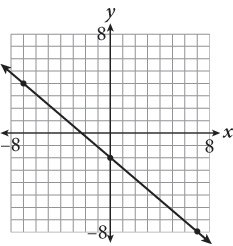What is the slope of the line shown in the graph?

• A. –2
• B.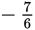• C.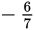• D. 2

Explanation:

C

Difficulty: Easy

Category: Heart of Algebra / Linear Equations

Strategic Advice: To find the slope of a line from its graph, either count the rise and the run from one point to the next, or choose two points that lie on the line and substitute them into the slope formula,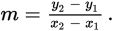Use whichever method gets you to the answer the quickest. Pay careful attention to negative signs.

Getting to the Answer: Using the points (0, –2) and (7, –8), the slope is: